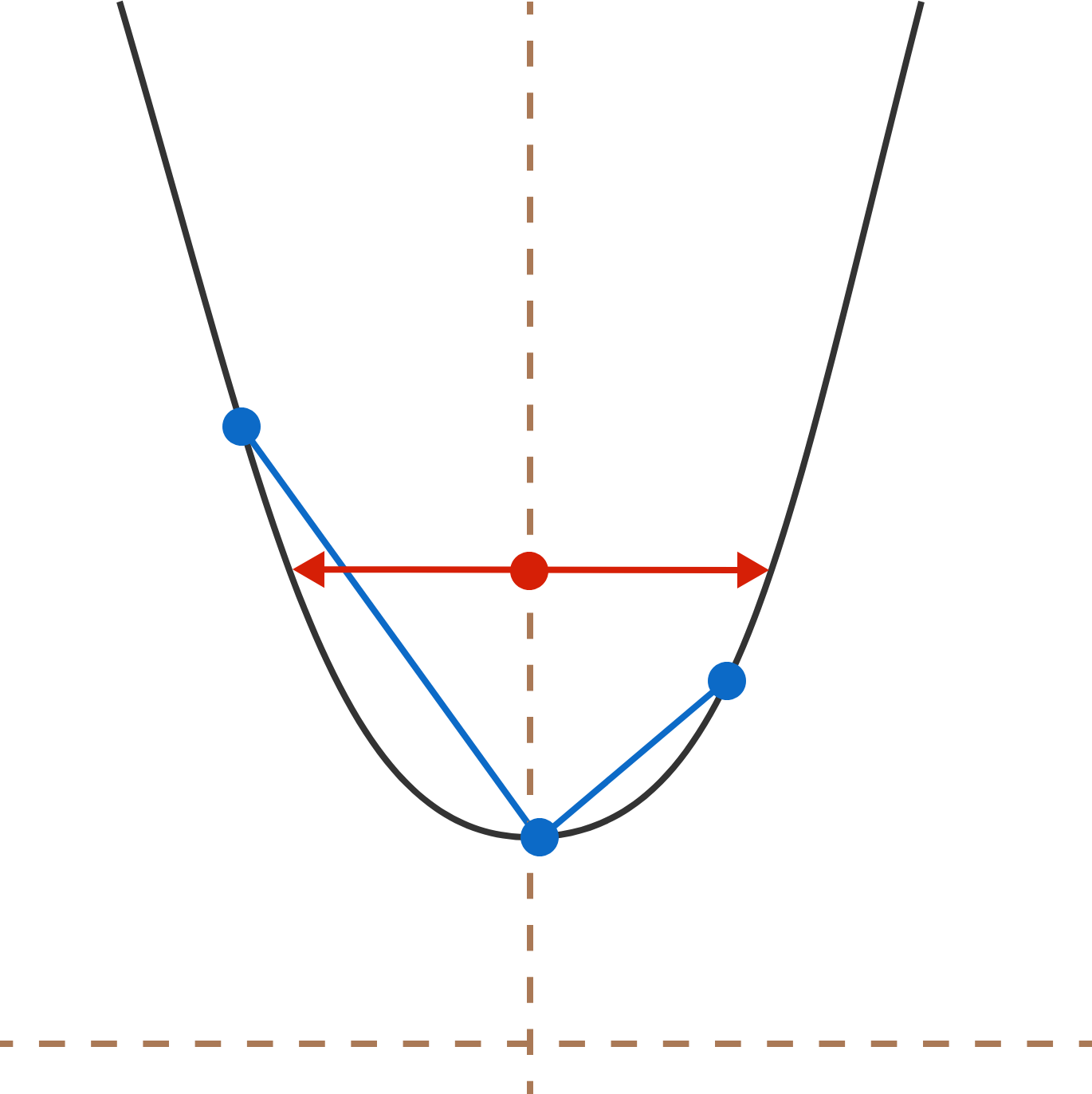# Strange Perpendicular Chords

Geometry Level 3Two mutually perpendicular chords are drawn from the vertex of parabola such that their lengths are $8$ and $27$. This is possible for only one distance between the parabola's focus and the directrix.

The length of latus rectum of such a parabola can be expressed as $\displaystyle \frac{a}{\sqrt{b}}$ where $a$ and $b$ are positive coprime integers and $b$ is square-free .

Enter the value of $a+b$.

Details and Assumptions:

• Latus rectum is the focal chord of a parabola which is perpendicular to the axis of the parabola.
×

Problem Loading...

Note Loading...

Set Loading...